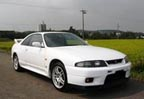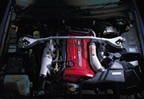Specifications

*R32 GTS-T
*R33 GTS25-T
*R34 GT-T
*R32 GTR
*R33 GTR
*R34 GTR
*R34 Z-Tune
*V35 GT350

Tuning Guides
*R32 GTS-T
*R33 GTS25-T
*R34 GT-T

*GTR
*RB30DET
*350GT

Videos
*R32
*R33
*R34
*V35/G35
*Other
*Project GTS25-T

Tech Resources
*Conversions
*Airflow meters
*Turbos
*Camshafts
*NVCS
*ATTESA
*HICAS

Projects
*Project GTS25T

Resources
*Videos
*Wallpaper
*Newletter
*Contact

Home > GTS-T Article# <!-- function roundTo2(n){ units = n * 100; units = Math.round(units); strUnits = " " + units; len = strUnits.length } function calckw1(form){ var hpvalue1 = parseFloat(form.hp1.value); kwvalue1 = hpvalue1 * 0.7457; roundTo2(kwvalue1); form.kw1.value = strUnits.substring(0, len - 2) + "." + strUnits.substring(len - 2, len); } function calchp2(form){ var kwvalue2 = parseFloat(form.kw2.value); hpvalue2 = kwvalue2 / 0.7457; roundTo2(hpvalue2); form.hp2.value = strUnits.substring(0, len - 2) + "." + strUnits.substring(len - 2, len); } function calckw3(form){ var psvalue3 = parseFloat(form.ps3.value); kwvalue3 = psvalue3 * 0.7355; roundTo2(kwvalue3); form.kw3.value = strUnits.substring(0, len - 2) + "." + strUnits.substring(len - 2, len); } function calcps4(form){ var kwvalue4 = parseFloat(form.kw4.value); psvalue4 = kwvalue4 / 0.7355; roundTo2(psvalue4); form.ps4.value = strUnits.substring(0, len - 2) + "." + strUnits.substring(len - 2, len); } function calchp5(form){ var psvalue5 = parseFloat(form.ps5.value); hpvalue5 = psvalue5 * 0.9863; roundTo2(hpvalue5); form.hp5.value = strUnits.substring(0, len - 2) + "." + strUnits.substring(len - 2, len); } function calcps6(form){ var hpvalue6 = parseFloat(form.hp6.value); psvalue6 = hpvalue6 / 0.9863; roundTo2(psvalue6); form.ps6.value = strUnits.substring(0, len - 2) + "." + strUnits.substring(len - 2, len); } function calcftlb(form){ var nmvalue = parseFloat(form.nm1.value); ftlbvalue = nmvalue / 1.356; roundTo2(ftlbvalue); form.ftlb1.value = strUnits.substring(0, len - 2) + "." + strUnits.substring(len - 2, len); } function calcnm(form){ var ftlbvalue = parseFloat(form.ftlb2.value); nmvalue = ftlbvalue / 0.7384; roundTo2(nmvalue); form.nm2.value = strUnits.substring(0, len - 2) + "." + strUnits.substring(len - 2, len); } function calckmh(form){ var mphvalue = parseFloat(form.mph1.value); kmhvalue = mphvalue / 0.621371192; roundTo2(kmhvalue); form.kmh1.value = strUnits.substring(0, len - 2) + "." + strUnits.substring(len - 2, len); } function calcmph(form){ var kmhvalue = parseFloat(form.kmh2.value); mphvalue = kmhvalue * 0.621371192; roundTo2(mphvalue); form.mph2.value = strUnits.substring(0, len - 2) + "." + strUnits.substring(len - 2, len); } //--> Nissan Skyline Conversions page

Nissan skyline coverter simply enter the unit in the appropriate box bellow and click the button to convert

Horsepower: HP
Kw

Kilowatts: Kw
HP

#### PS (Metric HP) to Kilowatts

PS (Metric HP): PS
Kw

Kilowatts: Kw
PS

#### PS (Metric HP) to Horsepower

PS (Metric HP): PS
HP

Horsepower: HP
PS

#### Nm to lb/ft (torque)

Newton metres: Nm
lb/ft

#### lb/ft to Nm (torque)

Foot Pounds: lb/ft
Nm

#### MPH to Km/h

Miles per Hour: MPH
Km/h

#### Km/h to MPH

Km per Hour: Km/h
MPH

Fly wheel power to wheel power
On the spec sheets for a factory car power is measured at the flywheel when you take a car to a chassis dyno power is measured at the wheels. This figure is always lower than the flywheel figure because the power is transferred into heat from the transmission, diff etc. The figures bellow are to be used as a rule of thumb real world figures may vary significantly.

 tranmision / driving wheels power lost or gained in kilowatts Manual rear wheel drive 60 Manual all wheel drive (GTR) 80 Automatic rear wheel drive 70